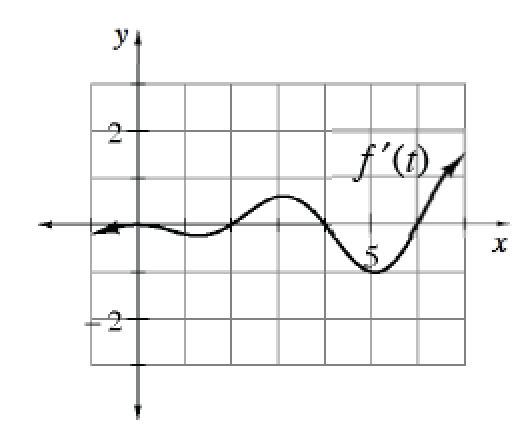### Home > APCALC > Chapter 11 > Lesson 11.2.3 > Problem11-71

11-71.

Multiple Choice: The graph at right represents $y = f^\prime(t)$. If $f(0) = 5$, then the value of $\frac { d } { d x } \int _ { 0 } ^ { 6 } f ^ { \prime } ( t ) d t$ is: Homework Help ✎

1. $0$

1. $5$

1. $2-\frac { \pi } { 2 }$

1. $4 −\frac { \pi } { 2 }$

1. $9 −\frac { \pi } { 2 }$If you evaluate the integral, do you get a function or a constant? What is the derivative of this function/constant?